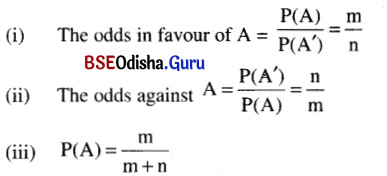# CHSE Odisha Class 12 Math Notes Chapter 6 Probability

Odisha State Board CHSE Odisha Class 12 Math Notes Chapter 6 Probability will enable students to study smartly.

## CHSE Odisha 12th Class Math Notes Chapter 6 Probability

Important terms:
(a) Random experiment: It is an experiment whose results are unpredictable.
(b) Sample space: It is the set of all possible outcomes of an experiment. We denote the sample space by ‘S’.
(c) Sample point: Each element of a sample space is a sample point.
(d) Event: Any subset of a sample space is an event.
(e) Simple event: It is an event with a single sample point.
(f) Compound event: Compound events are the events containing more than one sample point.
(g) Mutually exclusive events: Two events A and B are mutually exclusive if A ∩ B = φ (i.e. occurrence of one excludes the occurrence of other)
(h) Mutually exhaustive events: The events A1, A2, A3 ……. An are mutually exhaustive if A1 ∪ A2 ∪ A3 ∪ An = S.
(i) Mutually exclusive and exhaustive events.
The events A1, A2, A3 ……. An are mutually exclusive and exhaustive if
(i) A1 ∩ A2 = φ for i ≠ j
(ii) A1 ∪ A2 ∪ …… An = S.
(j) Equally likely events: Two events are equally likely if they have equal chance of occurrence.
(k) Impossible and certain events: φ is the impossible and S is the sure or certain event.
(i) Independent events: The events are said to be independent if the occurrence or non-occurrence of one does not affect the occurrence of non-occurrence of other.Probability of an event:
Let S be the sample space and A is an event then the probability of A is
$$P(A)=\frac{|A|}{|S|}=\frac{\text { No.of out comes favourable to } A}{\text { Total number of possible outcomes. }}$$
Note:
1. P(φ) = 0
2. P(S) = 1
3. P(A’) = P (not A) = 1 – P(A)
4. P(A) + P(A’) = 1

Odds in favour and odds against an event:
Let in an experiment is the number of cases favourable to A and ‘n’ is the number of cases not in favour of A thenIf A and B are any two events then
P(A ∪ B) = P(A) + P(B) – P(A ∩ B)
Note:
If A and B are mutually exclusive then
P(A ∪ B) = P(A) + P(B)
(∴ P(A ∩ B) = P(φ) – 0)

Conditional probability:
Let A and B are any two events and P(B) ≠ 0 then the conditional probability of A when B has already happened
P(A/B) = $$\frac{P(A \cap B)}{P(B)}$$
Note:
1. P(A ∩ B) = P(B) . P(A/B)
2. If A and B are mutually independent events then P(A/B) = P(A).
∴ P(A ∩ B) = P(A) . P(B)
3. If A and B are independent events then (i) A’ and B’ (ii) A’ and B (iii) A and B’ are also independent.
4. P(A1 ∩ A2 ∩ A3 ….. ∩ An) = P(A1) . P(A2/A1) . P(A3/A2 ∩ A1) ….. P(An/A1 ∩ A2 ∩ …. ∩ An-1)
5. Let A1, A2 ….. An are mutually exhaustive and exclusive events and A is any event which occurs with A1 or A2 or A3 … or An then
P(A) = P(A1) . P(A/A1) + P(A2) . P(A/ A2) + …….. + P(An) . P(A/An).
This is called the total conditional probability theorem.Baye’s theorem:
If A1, A2 …… An are mutually exclusive and exhaustive events and A is any event which occurs with A1 or A2 or A3 or …. An then
$$P\left(A_i / B\right)=\frac{P\left(A_i\right) \cdot P\left(A / A_i\right)}{\sum_{i=1}^n P\left(A_i\right) P\left(A / A_i\right)}$$